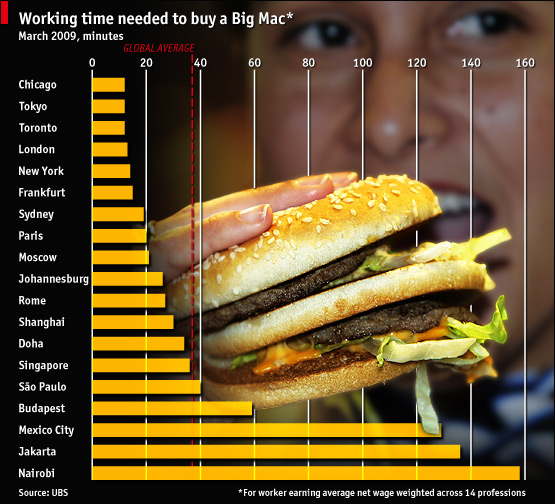Big Mac Index

•Purchasing power: An alternative Big Mac index | The Economist
• Big Mac index: exchange-rate theory | The Economist
• Overvalued/Undervalued Exchange rate
• If Swiss BigMac costs US\$6.81  (= SFr6.51 at an actual exchange rate of 0.96 to the dollar) (6.51/0.96)
• and US BigMac costs US\$4.20
• The Swiss franc is 62% (\$6.81/\$4.20=1.62) overvalued against the US dollar.
• The implied PPP = The exchange rate to equalize BigMac prices should be (SFr6.51/US\$4.20)=1.55
• Exchange rate of 0.96 is an overvaluation.  Exchange rate should be SFr1.55 per \$US.
• [1-(0.96-1.55)/1.55]*100 = +62 = the Swiss franc was overvalued against the dollar by 62%
• Big Mac Index - Wikipedia
• The Big Mac PPP exchange rate between two countries is obtained by dividing the price of a Big Mac in one country (in its currency) by the price of a Big Mac in another country (in its currency). This value is then compared with the actual exchange rate; if it is lower, then the first currency is under-valued (according to PPP theory) compared with the second, and conversely, if it is higher, then the first currency is over-valued.
• [1-((actual exchange rate)-(implied PPP))/(implied PPP)]*100
• Purchasing power parity - Wikipedia
• In essence, PPP theory predicts that changes in relative prices will result in a change in exchange rates
Since the growth rate of a country's money supply determines its likely future inflation rate, we can use information about the growth in money supply to forecast exchange rate movements.
While PPP theory seems to yield relatively accurate predictions in the long run, it does not appear to be a strong predictor of short-run movements in exchange rates covering time spans of five years or less.
PPP theory assumes away transportation costs and barriers to trade.
Global Business Today, CWLHill&TMcKaig, McGraw-Hill, 2009
• PPP = what the currency will buy in the county of issue
• Principle that exchange rates are set so that the prices of similar products in different countries are about the same
• Is a given currency over-valued or under-valued compared with another currency? Is it due to to market forces or government intervention? How long the average wage earner must work to earn enough money to pay for a Big Mac.
• Warning! - the actual purchasing power of the local currency may be much higher than that implied by exchange values